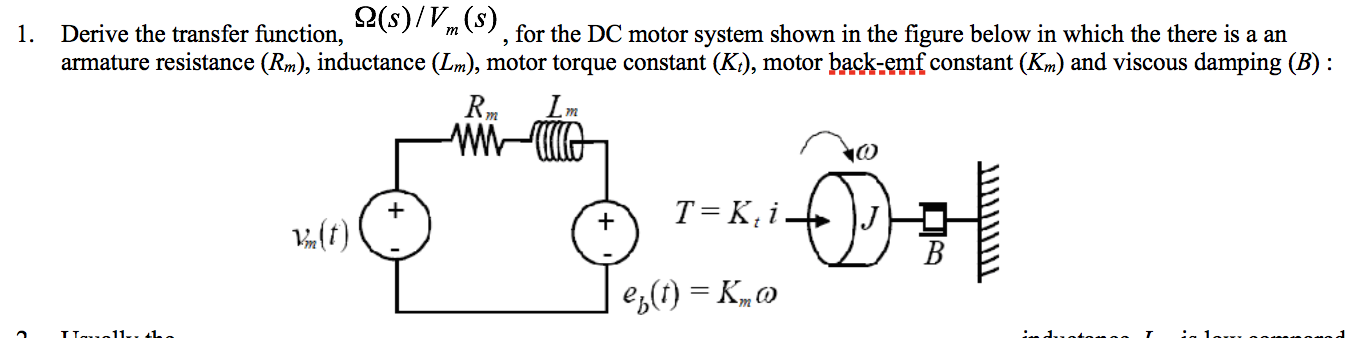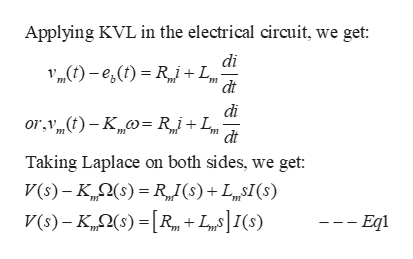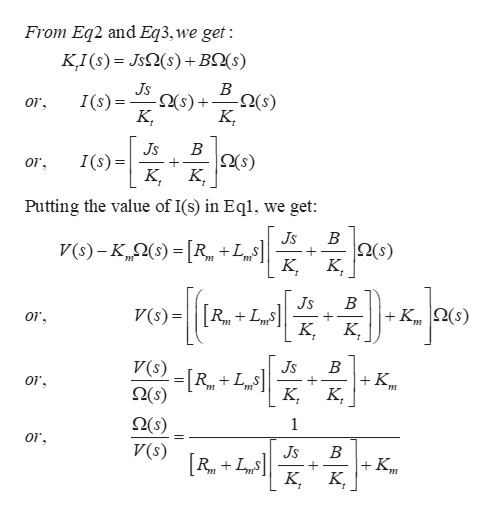# (s)/V(s)Derive the transfer function,armature resistance (Rm), inductance (Lm), motor torque constant (K), motor back-emf constant (Km) and viscous damping (B), for the DC motor system shown in the figure below in which the there is a an1.mmmT = K, i4(f)es() = K,

Question
4 viewshelp_outlineImage Transcriptionclose(s)/V(s) Derive the transfer function, armature resistance (Rm), inductance (Lm), motor torque constant (K), motor back-emf constant (Km) and viscous damping (B) , for the DC motor system shown in the figure below in which the there is a an 1. m m m T = K, i 4(f) es() = K, fullscreen
check_circle

Step 1

Equation of Electrical circuit :help_outlineImage TranscriptioncloseApplying KVL in the electrical circuit, we get di e()RiL dt di or,(t-K Rj+L dt Taking Laplace on both sides, we get: V(s) Ks)R()+LI(s) V(s)-K)RLI(s) -- Eqi fullscreen
Step 2

Now, the other equations:

Step 3

Further:...help_outlineImage TranscriptioncloseFrom Eq2 and Eq3, we get : KI(s) Js2(s)+BQ(s) В -2s) K Js -2(s) I(s) or K В (s) K, Js I(s)= or Putting the value of I(s) in Eql. we get B Js V(s)-K)R +L = K В Js 2(s) V(s) = or В K K Js V(s) or 2(s) 1 O(s) V(s) or В K Js LS K [R fullscreen

### Want to see the full answer?

See Solution

#### Want to see this answer and more?

Solutions are written by subject experts who are available 24/7. Questions are typically answered within 1 hour.*

See Solution
*Response times may vary by subject and question.
Tagged in

### Electrical Engineering